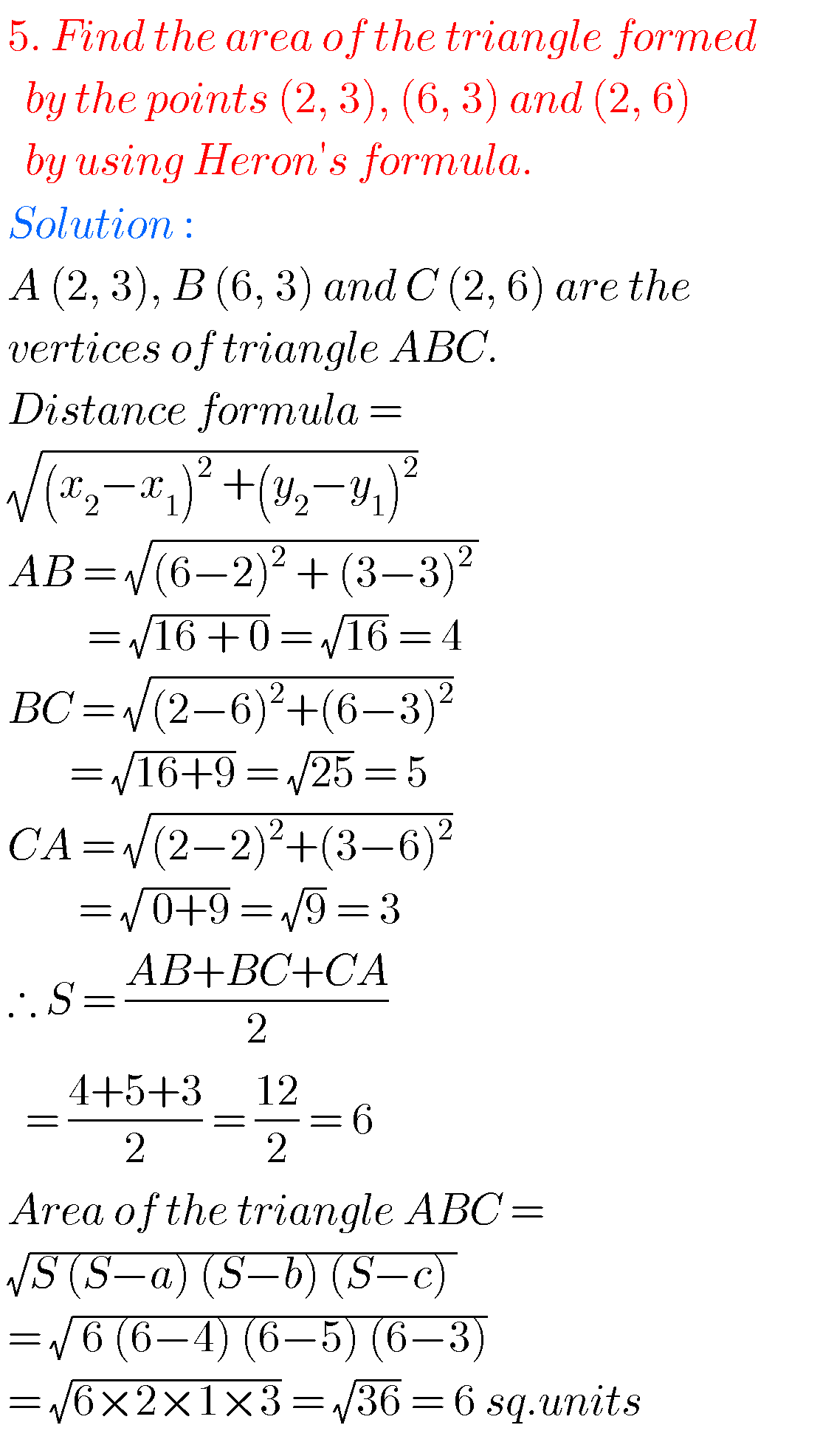## SSC solutions for Maths Coordinate Geometry class 10, AP & TS

SSC solutions for maths Coordinate Geometry Mathematics solutions for Coordinate Geometry class 10 SSC. Exercise 7.1, exercise 7.2, exercise 7.3, and exercise 7.4 solutions are given. Thsese solutions are very easy to understand. First study the textbook lesson Coordinate Geometry very well.  Observe the example problems and solutions given in the textbook. Observe the solutions …